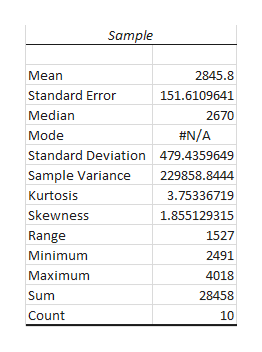# PrintTech, Inc. is introducing a new line of ink-jet printers and would like to promote the number of pages a user can expect from a print cartridge. A sample of 10 cartridges revealed the following number of pages printed. (Use  t Distribution Table.)           3,1042,7732,4912,5672,5092,8392,5002,5244,0183,133 a) What is the point estimate of the population mean? (Round your answer to 2 decimal places.)b) Develop a 98% confidence interval for the population mean.(Round your answers to 2 decimal places.)Confidence interval for the population mean is between ______and ______

Question

PrintTech, Inc. is introducing a new line of ink-jet printers and would like to promote the number of pages a user can expect from a print cartridge. A sample of 10 cartridges revealed the following number of pages printed. (Use  t Distribution Table.)

 3,104 2,773 2,491 2,567 2,509 2,839 2,500 2,524 4,018 3,133

a) What is the point estimate of the population mean? (Round your answer to 2 decimal places.)

b) Develop a 98% confidence interval for the population mean.(Round your answers to 2 decimal places.)

Confidence interval for the population mean is between ______and ______

check_circleExpert Solution
Step 1

The point estimate of the population mean is the sample mean. Therefore, the sample mean is obtained as follows:

Step 2

Descriptive statistics:

Step-by-step procedure to obtain the descriptive statistics using Excel:

• In Excel sheet, enter the data of Sample in any of the column.
• In Data, Select Data Analysis and Choose Descriptive Statistics.
• In Input Range Select variables Sample and Click Labels in First Row.
• Select Summary Statistics.
• Click Ok.

Output obtained using Excel is as follows:help_outlineImage TranscriptioncloseSample Mean 2845.8 Standard Error 151.6109641 Median 2670 #N/A Mode Standard Deviation 479.4359649 Sample Variance 229858.8444 Kurtosis 3.75336719 Skewness 1.855129315 1527 Range Minimum 2491 Maximum 4018 Sum 28458 Count 10 fullscreen
Step 3

From the output, the sample mean is 2,845.80. Thus, the point estimate of the population mean is 2,845.80.

b.

...

### Want to see the full answer?

See Solution

#### Want to see this answer and more?

Solutions are written by subject experts who are available 24/7. Questions are typically answered within 1 hour*

See Solution
*Response times may vary by subject and question
Tagged in

### Statistics